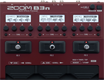# Electric Uncle Sam - Primus

Discussion in 'Zoom B3n' started by Kalmek, Nov 4, 2022.

1. Electric Uncle Sam - PrimusDevice: Zoom B3n
Firmware: 2.20

Name on device: E.Sam - P
Optimized for: Bass Amp

Effects chain:Trying to emulate the sound of Electric Uncle Sam from Antipop album. There may be additional synth in the recording hence the slight octave growl, which i tried to emulate via Z-Syn fx.
Preset was created for JB, which is also not the bass Les uses for this song i suppose.

Effect: "ZNR" (Dynamics), active - "yes"
"Detect" = EFXIN
"Depth" = 68
"Threshold" = 30
"Decay" = 0

Effect: "Bass Pre" (Overdrive / Distortion), active - "yes"
"Bass" = 5
"Middle" = 0
"Treble" = 6
"Volume" = 64

Effect: "Bass OD" (Overdrive / Distortion), active - "yes"
"Gain" = 48
"Tone" = 28
"Balance" = 46
"Volume" = 88

Effect: "High EQ" (Filter), active - "yes"
"Type" = SHELF
"Frequency" = 4600
"Gain" = 0.0
"Volume" = 80

Effect: "Z-Syn" (Sfx), active - "yes"
"Frequency" = 5
"Range" = 6
"Decay" = 12
"Resonance" = 11
"Wave" = SAW
"Tone" = 8
"Balance" = 32
"Volume" = 88
"Frequency" = 2
"Range" = 8
"Decay" = 72
"Resonance" = 18
"Wave" = SAW
"Tone" = 7
"Balance" = 80
"Volume" = 80
"Parameter set" = A
Patch Volume: 100